# Number Bonds to 100

Number Bonds to 100• Number Bonds to 100 are pairs of numbers that add together to make 100.
• To find the number bond that pairs with 28 to make 100, we think of the number that we add to 28 to make 100.
• We can add 2 to 28 to make the next multiple of 10, which is 30.
• Now from 30, we just add 70 to make 100.
• In total we have added 70 + 2.
• 28 and 72 are number bond pairs that add to make 100.

To find a number bond to 100, first add on to the next multiple of 10 and then add on the required multiple of 10 to get to 100.• In this number bond example, we are asked which number pairs with 64 to make 100.
• First we add 6 to get to the next multiple of ten, which is 70.
• From 70, we need to add 30 more to make 100.
• Our number bond that pairs with 64 to make one hundred is 36.Supporting Lessons

#### Number Bonds to 100#### Number Bonds to 100 Interactive Questions

Number Bonds to 100 – Multiples of 10: Interactive Questions

Number Bonds to 100: Interactive Questions

# What are Number Bonds to 100?

Number Bonds to 100 are pairs of numbers that add together to make 100.

To find a number bond to 100, first add on to reach the next multiple of ten and then add the multiples of ten needed to get to 100.

When learning or teaching number bonds to 100, we must first make sure that we know our number bonds to ten: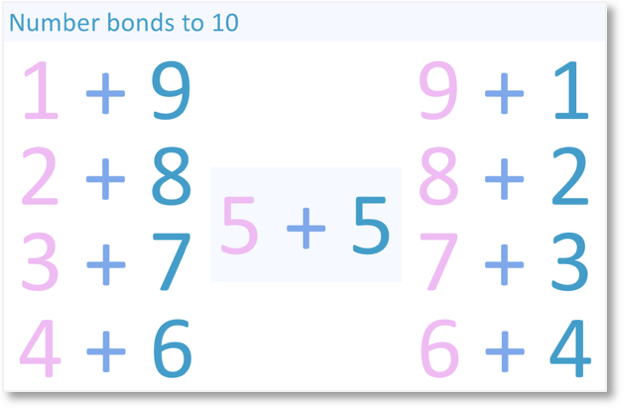We can use our number bonds to ten to help us learn our multiples of 10 which are number bonds to 100 , which are the pairs of multiples of ten that add to make 100.

The multiples of 10 which are number bonds to 100 are the same as the number bonds to ten but each number is multiplied by 10.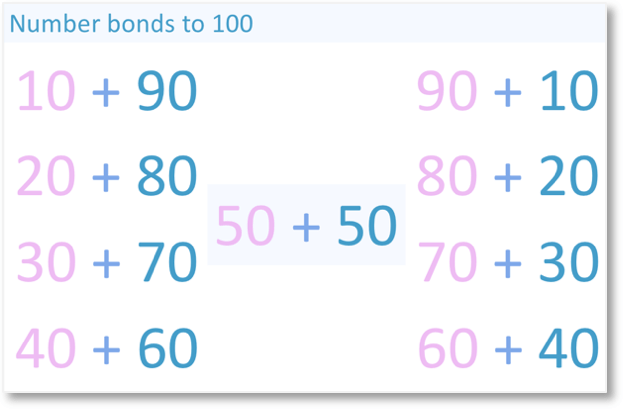We can then use both of these number facts to find all combinations of the number bonds to 100.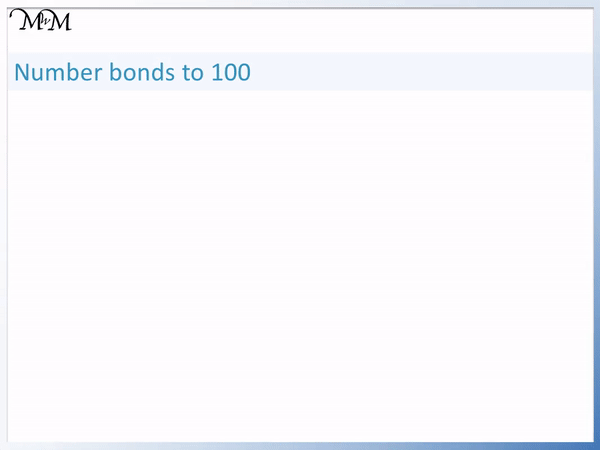When teaching the number bonds to 100, it is best to first learn the multiples of 10 that add to make 100.

It is easiest to remember these as pairs of numbers, which are:

10 and 90, 20 and 80, 30 and 70, 40 and 60, along with 50 and 50.

To find any number bond to 100, count up to the next multiple of ten and use the multiple of 10 number bonds shown above to reach 100.

Here is an example. Find the number bond that pairs with 28 to make 100:The example is actually asking us what do we need add to 28 to equal 100?When teaching your child number bonds, you can begin by drawing a simple number line.

We draw a line starting at 28 and ending at 100.

We want to get from 28 to 100 using addition.

We can do this by carrying out two numerical addition jumps.We begin by adding on from 28 to get to the next ten.

The next multiple of ten after 28 is 30.

To get from 28 to 30, we add 2.

We know this since 28 ends in 8 and the number bond to 10 that pairs with 8 is 2.Our next addition jump will be from 30 to 100.

To get from 30 to 100, we add 70.In total, we have added 2 and 70.

So, in total we have added 72.

Therefore the number bond that pairs with 28 to make 100 is 72.

28 + 72 = 100

Here’s another example. Find the number bond that pairs with 45 to make 100: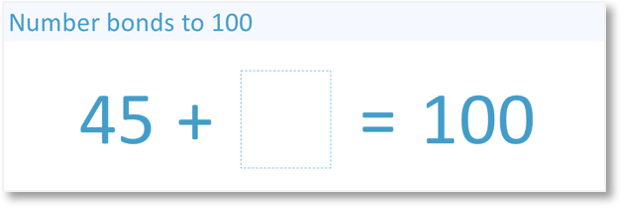Remember that a number bond to 100 is the addition sum that takes us from our original number up to 100. The question is asking us what number we add to 45 to make 100.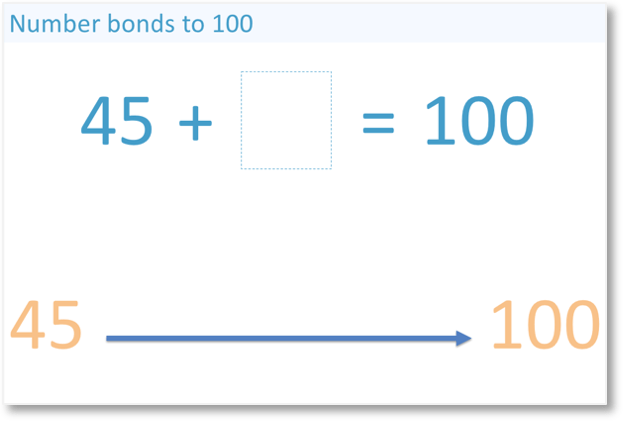We want to get from 45 to 100 using addition.First, we perform an addition jump from 45 to the next multiple of ten, which is 50.

To get from 45 to 50, we add 5.

Again we should know this addition fact from our number bonds to 10.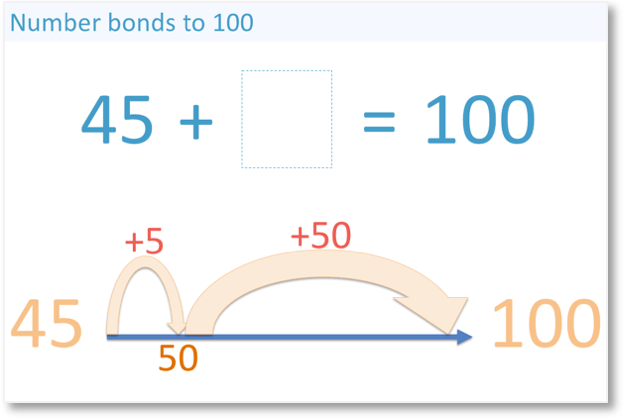To get from 50 to 100, we add 50.

When teaching number bonds, explain the link between these simple multiples of ten that pair to make 100 and the original number bonds to 10.Altogether, we have added 5 and 50.

So, we have added 55 in total to make 100.

Therefore our number bond that pairs with 45 to make 100 is 55.

45 + 55 = 100.

When teaching number bonds to 100, it is helpful to have a number line to hand in order to visualise the first jump to the next multiple of 10 and then the second jump to 100.Now try our lesson on Addition Facts to 20 where we learn how to work out and remember the answers to pairs of additions up to 20.